# R things I, and perhaps others, forget

This is a developing miscellany of the things that I seem to keep forgetting about R. What I used to call in my student days “teflon coated facts”!

Chris Evans https://www.psyctc.org/R_blog/ (PSYCTC.org)https://www.psyctc.org/psyctc/
2023-06-16

# Clipping ggplot plot axes

I always forget how to do this, I guess it’s not something I need very often, but when I do it seems very hard to find the answer by searching as the obvious words to search on seem to take me to controlling the axis itself, not these little expansions/extensions. So here’s how to do it.

The default is that ggplot adds a small extension to the axes. So here is the default for a silly little plot. With rather crude annotation to show what I mean.

Show code
``````seq(1, 4, length = 500) %>%
as_tibble() %>%
rename(x = value) %>%
mutate(y = x) -> tibDat

ggplot(data = tibDat,
aes(x = x, y = y)) +
geom_point() +
annotate(geom = "label",
x = 1.3, y = 1.8,
label = "Left hand x margin here",
size = 8) +
geom_segment(x = 1.1, xend = .92,
y = 1.74, yend = 1,
arrow = arrow(angle = 30, length = unit(0.02, "npc"),
ends = "last", type = "open")) +
annotate(geom = "label",
x = 1.85, y = 1.1,
label = "Left hand y margin here",
size = 8) +
geom_segment(x = 1.4, xend = 1,
y = 1.1, yend = .92,
arrow = arrow(angle = 30, length = unit(0.02, "npc"),
ends = "last", type = "open")) +
theme(axis.text.x = element_text(size = 28),
axis.text.y = element_text(size = 28),
axis.title.x = element_text(size = 28),
axis.title.y = element_text(size = 28))``````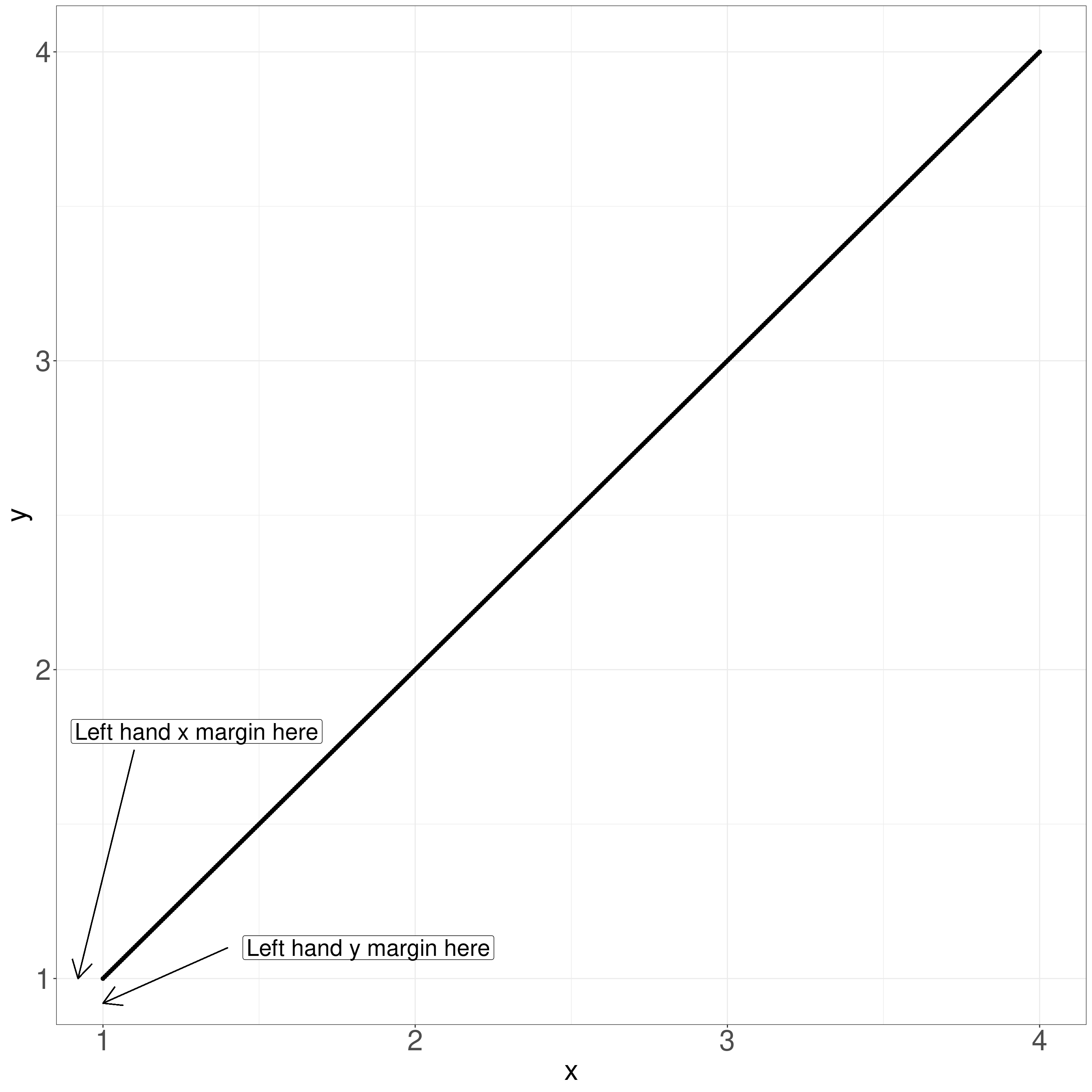Here I use `xlim(c(1.5, 3.5))` and `ylim(c(1.5, 3.5))` to shorten the axes…

Show code
``````ggplot(data = tibDat,
aes(x = x, y = y)) +
geom_point() +
xlim(c(1.5, 3.5)) +
ylim(c(1.5, 3.5)) +
theme(axis.text.x = element_text(size = 28),
axis.text.y = element_text(size = 28),
axis.title.x = element_text(size = 28),
axis.title.y = element_text(size = 28))``````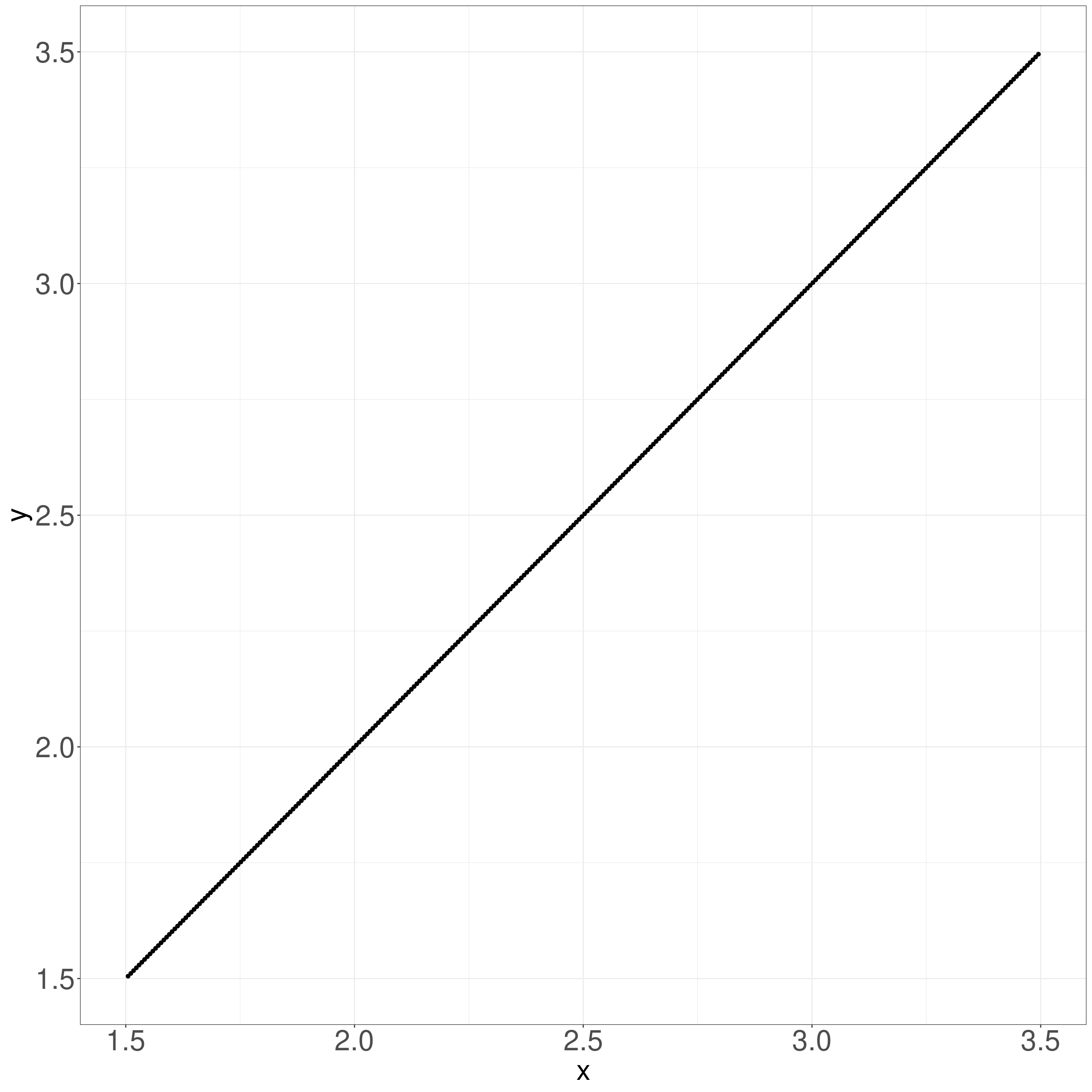Of course a lot of points have been dropped to pull the plot back to these limits. But there is still this margin on the axes. The trick is to add

``````  scale_x_continuous(expand = c(0, 0)) +
scale_y_continuous(expand = c(0, 0))``````
Show code
``````ggplot(data = tibDat,
aes(x = x, y = y)) +
geom_point() +
scale_x_continuous(expand = c(0, 0)) +
scale_y_continuous(expand = c(0, 0)) +
theme(axis.text.x = element_text(size = 28),
axis.text.y = element_text(size = 28),
axis.title.x = element_text(size = 28),
axis.title.y = element_text(size = 28))``````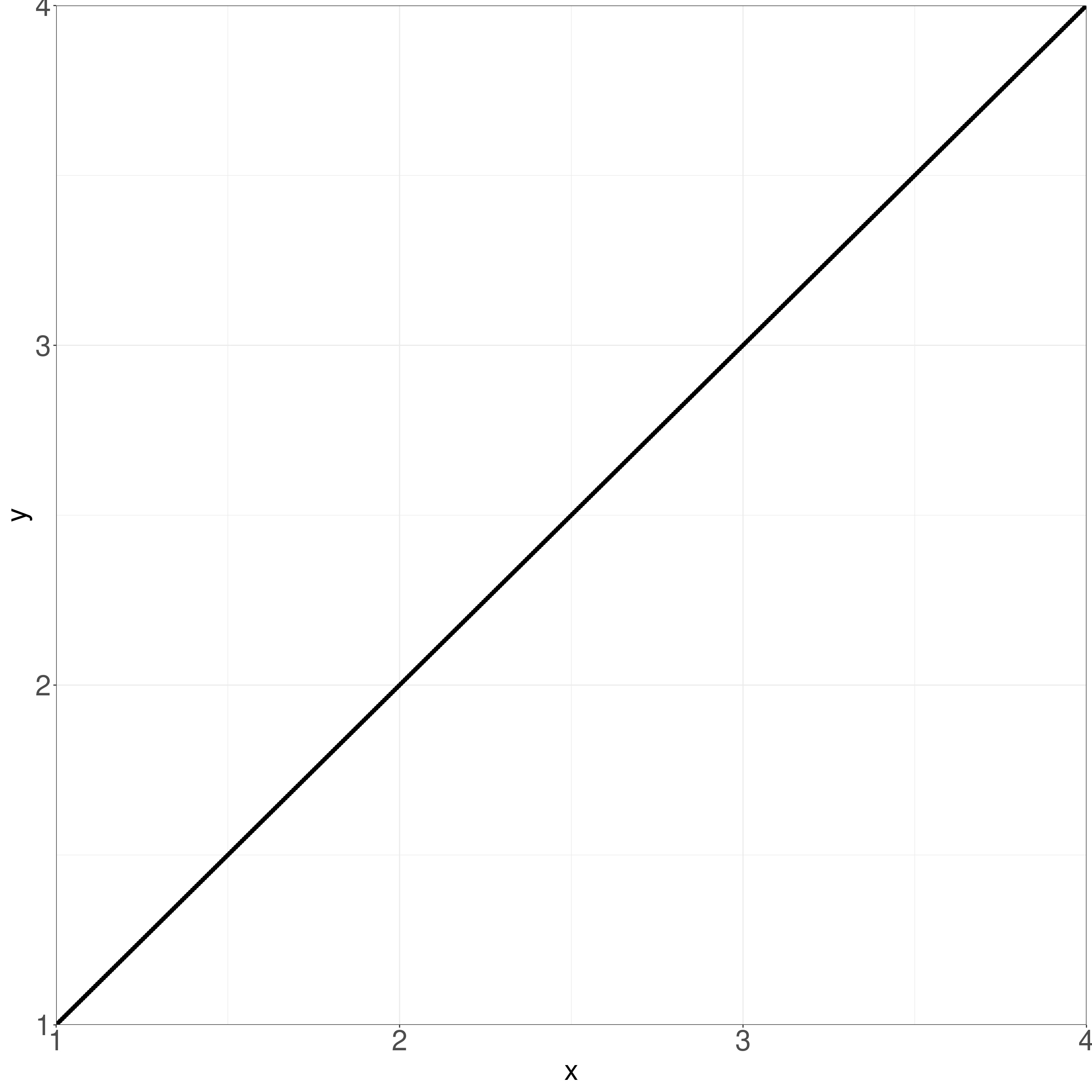This is not the same as `expand_limits()`

Show code
``````ggplot(data = tibDat,
aes(x = x, y = y)) +
geom_point() +
expand_limits(x = c(1, 4)) +
theme(axis.text.x = element_text(size = 28),
axis.text.y = element_text(size = 28),
axis.title.x = element_text(size = 28),
axis.title.y = element_text(size = 28))``````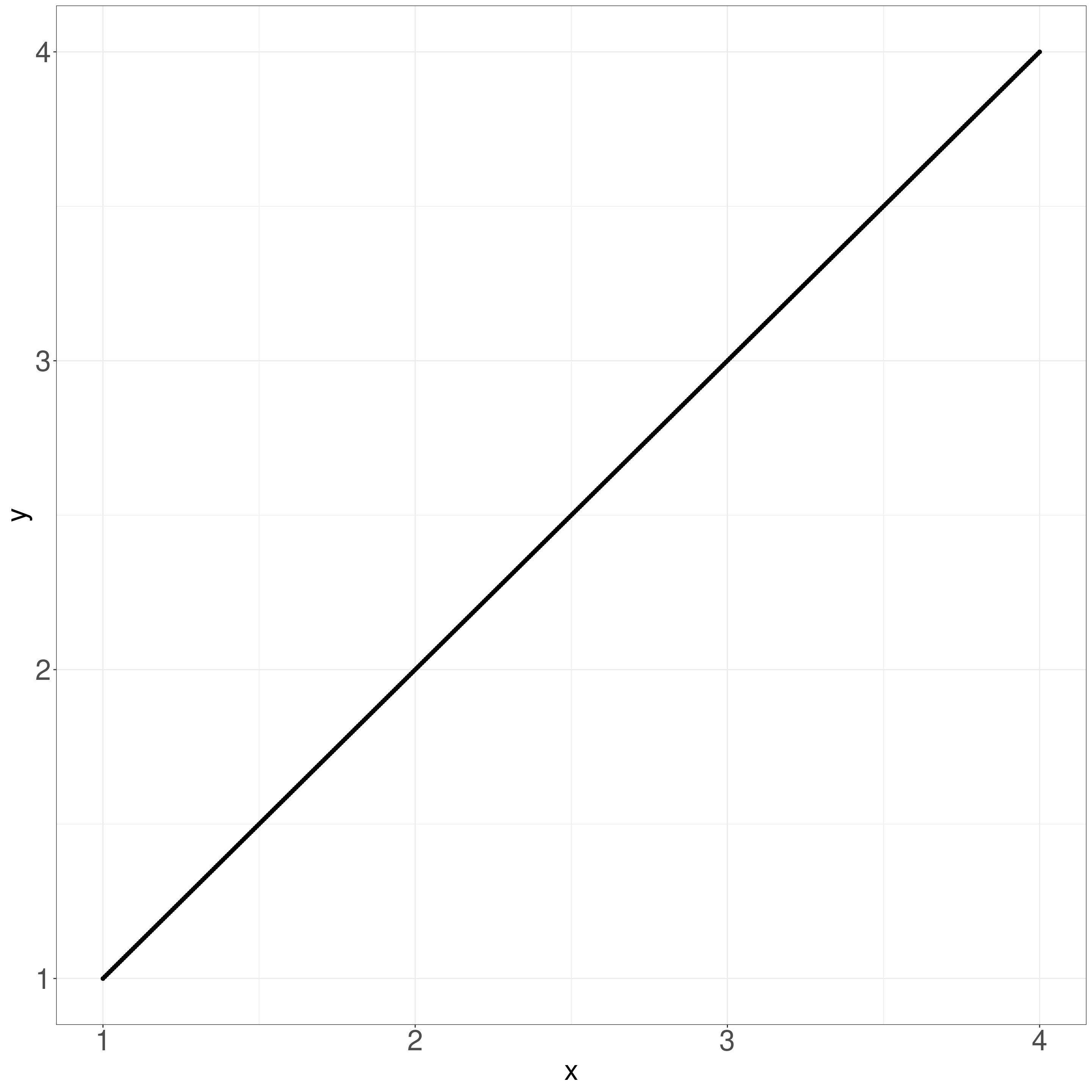But you can use `expand_limits()` to create space on the plot. Here I’ve used `scale_x_continuous(breaks = 1:4)` to stop the x axis getting labelled up to 6.

Show code
``````ggplot(data = tibDat,
aes(x = x, y = y)) +
geom_point() +
expand_limits(x = c(1, 6)) +
annotate(geom = "label",
x = 4.3, y = 3,
label = "This allowed me to put\na label in here\nwhere I can put in\nlots of drivel\nand other nonsense",
hjust = 0,
size = 10) +
scale_x_continuous(breaks = 1:4) +
theme(axis.text.x = element_text(size = 28),
axis.text.y = element_text(size = 28),
axis.title.x = element_text(size = 28),
axis.title.y = element_text(size = 28))``````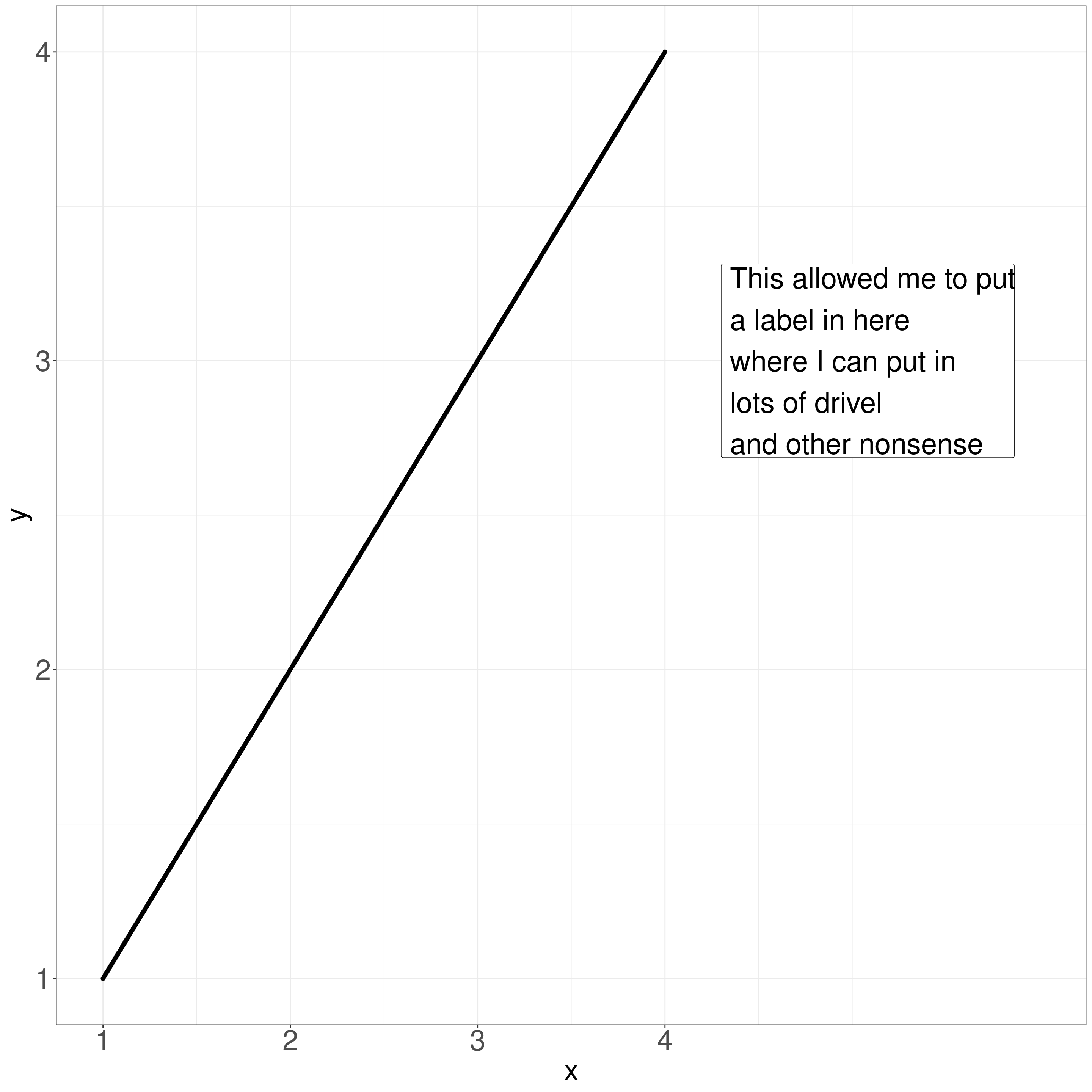# Putting citations and references into Rmarkdown/Distill documents

This is something I do from time to time and I’m not claiming this is the best way to do it but it worked for me doing the post Jacobson #1 which, as is my usual, I did in Rstudio. This may not, perhaps probably won’t work if you using a different editor/environment. This also assumes you are using Zotero as your bibliographic database manager … which I do because it’s open source and excellent. You need the Better Bibtex for Zotero plugin for Zotero. That’s what the rbbt package connects to when it’s finding references.

I installed the rbbt library. It’s not in CRAN so:

``remotes::install_github("paleolimbot/rbbt")``

That adds addins to the Rstudio addin menu and if you use the “Insert Zotero Citation” you get into the usual Zotero plugin lookup to select the reference(s) you want. The “Insert Zotero bibliography from Zotero Selection” puts the bibliography in at the end of the document so you generally want to end the document with a top level heading “References”

The really neat bits are that the plugin will collect just the references you cited into a bib format bibliography for you. To get that you put this

``bibliography: tmpBib.bib``

in the yaml header of the Rmarkdown document. You can call the bib file anything you like of course but it has to be in the same directory as the source file. (So for a distill blog post it goes in the directory for the post, not in the project root. Don’t worry if you don’t use distill as life’s simple then: just keep the bib file with the Rmd file.)

To get this to work, first make sure you have saved your Rmarkdown document since you last added a citation and then use the third (and last) addin call created by the rbbt package: “Update bibliography for current document from Zotero” which does what that says and tells you in the console tab how many references it has added.

Working like this allows you to use all the Rmarkdown citation tricks like ommitting the author(s) names, adding a reference so it will appear in the reference list despite not being cited in the document. See 4.5 Bibliographies and citations in bookdown.org for more on that.

The final neat bit is that one of these strengths is that you can set the formatting for the citations and reference list. I tend to use, despite a bit of grinding of teeth at the rigidity of it all, the APA rules for that but you can get a wide range of csl format files for different journals and rules. That is set by having this in the yaml header.

``csl: apa.csl``

You can get csl files from https://github.com/citation-style-language/styles.

# Update history of this post

* 16.vi.23: Started this just with the first two headings for clipping ggplot axes and referencing in Rmarkdown. Updated with Distill bit 9.viii.23

### Reuse

Text and figures are licensed under Creative Commons Attribution CC BY-SA 4.0. The figures that have been reused from other sources don't fall under this license and can be recognized by a note in their caption: "Figure from ...".

### Citation

`Evans (2023, June 16). Chris (Evans) R SAFAQ: R things I, and perhaps others, forget. Retrieved from https://www.psyctc.org/R_blog/posts/2023-06-15-r-things-i-and-perhaps-others-forget/`
```@misc{evans2023r,